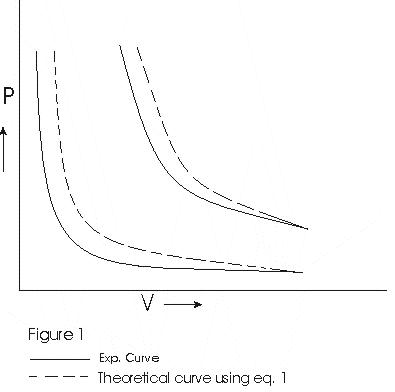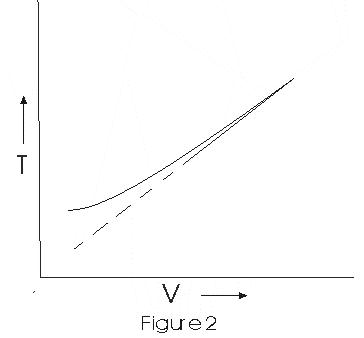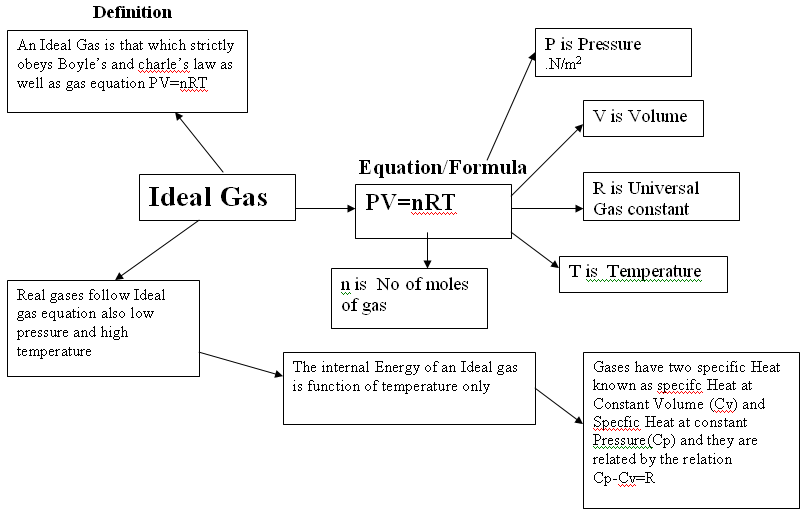# Behaviour Of Gases

## Gas Laws

Gas laws are study of any two of quantities like pressure, volume and temperature, when the third is kept constant

A. Boyle's law

• "At constant temperature, the volume of a given mass of gas is inversely proportional to pressure." Thus
V ∝ 1/P
Or, PV = constant(1)
• If P1, V1are initial pressure and volumes and P2, V2be final values then,
P1V1= P2V2
• Graph between P and V at temperature T1 and T2 such that T1<T2 are shown below,• Graph above shows that Boyle's law is strictly not obeyed by gases at all values of P and T but it obeys this law only at low pressure and high temperature i.e., at law density
B. Charle's Law
• Charle's Law is stated as follows :
"When pressure of a gas is constant the volume of a given mass of gas is directly proportional to its absolute temperature".
V/T = Constant(2)
• Graph between V and T isThis graph shows that experimental graph deviates from straight line. Theoretical and experimental graphs are in agreement at high temperature.

## Ideal gas equation

• We can combine Boyle' law eqn (1) and Charle's' law eqn (2) in to a single eqn i.e.,
PV/T = Constant(3)
If n moles is the mass of gas then we write
PV = nRT(4)
where, n is number of moles of gas, R=NAKB is the universal constant known as gas constant and T is the absolute temperature.
• A gas satisfying eqn (4) at all values of pressures and temperatures is said to be an ideal gas
now no of moles of gas
n = m/M = N/NA
where
m - mass of gas containing N molecules
M - molar mass
From this,
P = ρRT/M
ρ - mass density of gas.## Dalton law of partial pressures

• Consider a mixture of non-interacting ideal gases with n1 moles of gas 1,n2 of gas 2 and so on
• Gases are enclosed in an enclosure with volume V, temperature T and pressure P.
• Equation of state of mixture
PV = (n1+ n2)RT
orP = n1RT/V + n2RT/V + - - - -
= P1 + P2 + - - --
where,
P1 = n1RT/V
is pressure the gas 1 would exert at same V and T if no other gases were present in the enclosure. This is know as law of partial pressure of the gases.
• The total pressure of mixture of ideal gases is sum of partial pressures of individual gases of which mixture is made of.